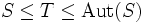# Almost simple group

## Definition

### Symbol-free definition

A group is said to be almost simple if it satisfies the following equivalent conditions:

### Definition with symbols

A group$G$ is said to be almost simple if it satisfies the following equivalent conditions:

• There is a simple non-abelian group$S$ such that$S \le T \le \operatorname{Aut}(S)$ for some group$T$ isomorphic to$G$.
• There exists a normal subgroup$N$ of$G$ such that$N$ is a simple non-abelian group and$C_G(N)$ is trivial.
This article defines a group property: a property that can be evaluated to true/false for any given group, invariant under isomorphism
View a complete list of group properties
VIEW RELATED: Group property implications | Group property non-implications |Group metaproperty satisfactions | Group metaproperty dissatisfactions | Group property satisfactions | Group property dissatisfactions
This is a variation of simplicity|Find other variations of simplicity | Read a survey article on varying simplicity

## Facts

• Automorphism group of simple non-Abelian group is complete
• Almost simple not implies simple or complete: An almost simple group need not be either simple or complete: in other words, it can be properly sandwiched between a simple group and its automorphism group.
• Symmetric groups are almost simple: For$n \ge 5$, the symmetric group on$n$ letters is almost simple. Note that for$n \ne 6$, it is in fact the whole automorphism group.